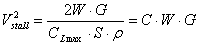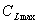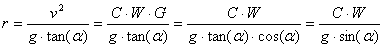# A Note on Steep Turns and Wind

I wrote “Flying around Manhattan” about a month before the tragic crash of Yankee pitcher Cory Lidle into a Manhattan building, the first collision of a general aviation fixed-wing airplane with a New York skyscraper since the dawn of aviation.  On the fateful day, an east wind was blowing at 14G20 knots.  The event made me wonder about radii of steep turns and how wind affects them.  So I engaged in a bit of math:

We’ll do everything – in very un-American fashion – in metric units (the SI system).  To convert knots to meters/second, we have m/s = knots × 0.5144444.

We’re interested in level turns which means that, whatever the bank angle, the vertical component of lift must be 1 G, or an acceleration of g = 9.80665 m/s.  A bank angle of α° gives a horizontal component of lift equal to g × tan(α) – at bank (straight and level), the horizontal component of lift is g × tan(0) = 0, a bank angle of 45° gives a horizontal component of lift equal to g × tan(45°) = 1 g, and at 60°, the horizontal component of lift is g × tan(60°) = 1.7 g.

The horizontal component of lift will pull us through the turn.  In a steady, level turn, the horizontal component of lift equals the centripetal acceleration, a, which is given by a = ω²r = v²/r, where ω (Greek omega) is the angular velocity and r is the radius of the turn; v is the linear speed.

So, if we’re flying at a speed of v m/s and we make a level banked turn of α°, we have v²/r = g × tan(α).  Solving for r, the radius of the turn, we get

r = v²/(g × tan(α))

And, if we fill in all the constants and do the conversion from knots into m/s (0.51) and meters into feet (3.28), we get:

f = 3.28 × (0.51 v)²/(9.81 × tan(α)) = 0.0885 k² / tan(α)

where f is the turn radius in feet, k is the speed in knots and α, the bank angle in degrees.

Let’s give that some meaning.  Here’s a table with various speeds (in knots) and bank angles (in degrees), giving the turn radius (in feet):

 Turn radius in feet Bank angle in degrees 20 30 40 50 60 70 Speed in knots 60 876 552 380 267 184 116 70 1192 751 517 364 250 158 80 1557 981 675 475 327 206 90 1970 1242 855 602 414 261 100 2433 1534 1055 743 511 322 110 2943 1856 1277 899 619 390 120 3503 2208 1519 1070 736 464 130 4111 2592 1783 1256 864 545 140 4768 3006 2068 1456 1002 632

So, if you can reliably fly a 45°-degree banked turn, at 70 knots you can do a 180 in about 600 feet (remember that the radius of the turn is half the diameter), while at 110 knots, you’re going to need more than 2000 feet.  That’s a dramatic difference!

At a bank angle of 60°, you’ll pull 2 Gs, and you need 500 feet at 70 knots and 1020 at 100 knots.  Note that speed affects the turn radius much more than bank angle.

# Optimizing the turn

Now we can ask ourselves, what is the optimum combination of speed and bank angle for a minimum-radius turn?  We’d have to slow down as much as possible and bank as much as possible.  But, if you go slower, the maximum bank angle you can use goes down because you’ll stall if you pull too many Gs.  Stall speed is given by the equation(adapted from Kershner’s Advanced Pilot Manual).  Since, S and ρ are constants, we’ll just replace them by C.  For a given aircraft, the denominator in the equation is a constant, so stall speed is proportional to √W, the weight of the aircraft and the number of Gs you pull in the turn (which, effectively, is a multiplicator for the weight of the aircraft).  In horizontal flight, G = 1, so the formula reduces to the one given by Kershner.

If the stall speed of your aircraft at some given weight is 50 knots, for example, and you pull a 60°, 2G turn, stall speed becomes, or 1.41 × 50 = 70 knots.  Now, assume you choose a safe minimum flying speed that is, say, 1.3 times the stall speed.  This minimum speed will grow as the bank angle increase – because you want to continue to fly at 1.3 times the stall speed.

G relates to bank angle α (in a level, constant-speed turn) like this: G = 1/cos(α).  We’ll substitute this in the stall speed equation and then substitute that result in the turn radius equation, giving:Just two variables remain, r, the turn radius, and α, the bank angle; sin(α) increases steadily with increasing bank angle, albeit with diminishing speed, to reach its maximum at a 90° angle.  The minimum radius turn is thus achieved at a 90° bank.  But you’ll be pulling infinitely many Gs and your airspeed will have to be astronomical to prevent the stall.  No aircraft is built to do that.  But the fact remains that the steepest possible turn gives the best turn radius.

The maximum steepness of the turn depends on the number of Gs it causes and thus on the structural forces the aircraft can withstand.  The Cessna R182 I fly, for example, is certified for a maximum of 3.8 Gs, which corresponds to a bank angle of 75°.  The stall speed in level flight is 55 knots (forward CG).  At 3.8 Gs, that becomes 55· √3.8 = 107 knots.  The radius of the resulting turn has a radius of just 273 feet (a diameter of 546 feet).

Should we try that?  Better not!

Banks of more than 60 degrees count as aerobatic maneuvers – you can’t do them without a parachute and you certainly can’t (and shouldn’t) do them over the East River.

So here’s what I do:

At a 60-degree bank, our calculations give: G force is 2, minimum safe airspeed becomes 55·1.3·√2 = 101 knots; turn radius is 0.0885·101² / tan(60°) = 522 feet: diameter 1044 feet.  I feel perfectly comfortable with this.  In fact, I usually fly these 60-degree banked turns between 100 and 120 knots and that gives me plenty of room to turn just south of (i.e., before reaching) Roosevelt Island.

The POH for the R182 gives a significant difference between indicated and calibrated airspeeds for the stall:  with forward CG, indicated is 39 KIAS, while calibrated is 54 KCAS; with rearward CG it’s 41 KIAS and 55 KCAS.  For the calculations above we obviously need true airspeed, and calibrated is closer to that than indicated.  But when you fly, you have to rely on indicated airspeed.  You and I are likely to monitor the airspeed before the turn, while still straight and level at or above 100 KIAS.  At these speeds, and in level flight, the difference between indicated and calibrated airspeed is small.

The POH for my R182 (1981) gives a stall-speed table for bank angles of 0°, 30°, 45°and 60° and both indicated and calibrated airspeeds.  For flaps-up, forward CG, 3100 lbs, Figure 5-3 gives:

 Bank KIAS KCAS KIAS/KCAS 0° 41 55 .75 30° 44 59 .75 45° 49 65 .75 60° 58 78 .74

Note that the error in indicated airspeed is the same, for every entry in the table.  My hypothesis is that the error is almost entirely a function of angle of attack and, at the stall, this, of course, is precisely the critical angle of attack and, therefore, the same for each table entry.

Using the airspeed calibration chart, which is for level flight, 58 knots indicated corresponds to 64 knots calibrated, which isn’t quite enough to prevent a stall even at 45 degrees of bank.  Again, this emphasizes the need for a healthy safety margin and for knowing what you’re doing before attempting any kind of flying anywhere near the performance limits of your airplane.

# Now for the Wind

While you turn, a wind from the side is going to widen or narrow the turn, depending on whether you turn with the wind (bad idea in the East River) or against it (good idea).  How much you’re going to be displaced by it depends on how long you spend in the turn.   Time is distance divided by speed, so the time in a 180° turn is πr/v.  If we use meters, and meters/second, we get time in seconds.  Here’s the table again, with the number of seconds to complete a 180 (please note that some combinations of bank angle and speed don’t make sense – there are probably few aircraft that can do a level, 70° banked turn at 60 knots without falling out of the sky):

 Seconds to complete a 180 Bank angle in degrees 20 30 40 50 60 70 Speed in knots 60 27 17 12 8 6 4 70 32 20 14 10 7 4 80 36 23 16 11 8 5 90 41 26 18 12 9 5 100 45 29 20 14 10 6 110 50 31 22 15 10 7 120 54 34 24 17 11 7 130 59 37 26 18 12 8 140 63 40 27 19 13 8

Now, isn’t it interesting that, the faster you go, the longer the turn takes?

Using the conversion above from knots to m/s, we see that a 10-knot wind is 10 × 0.51 or 5 m/s, so, if you’re exposed to it for 10 seconds, you’ll be pushed some 50 meters or 150 feet by the wind.  A k-knot wind will increase or decrease the diameter of an s-second 180 by k × s × 1.688 feet.

If you make a 45-degree banked turn at 110 knots with a wind of 14 knots in your back, the diameter of the turn becomes 2000 + 14 × 18 × 1.688 feet = 2425 feet.  If you turn against the wind, it’d be 2000 – 14 × 18 × 1.688 feet = 1575 feet; that’s just two thirds, not bad.

Another thing to notice from the table is that, the slower you go, the shorter the turn takes, so the shorter you’re exposed to wind pushing you off course.

So imagine doing a slightly shallower 40-degree banked turn, but now at 80 knots with a wind of 14 knots in your back, the diameter of the turn becomes 1350 + 14 × 16 × 1.688 feet = 1728 feet.  If you turn against the wind, it’d be 1350 – 14 × 16 × 1.688 feet = 972 feet; that’s about half and it’s quite a bit better than the steeper turn at 110 knots.

So bear this in mind:  Speed matters much more than bank angle if you need to make a tight turn, so slow down and don’t overdo the bank angle to keep you from stalling, drop some flaps if necessary.  If there is more than a few knots wind, think about which way you should turn to take advantage of it.  Wind can make a significant difference.

And, again, don’t practise those steep turns anywhere near something hard and unyielding.

December 2006, Sape Mullender